Math Calculators, Lessons and Formulas

It is time to solve your math problem

mathportal.org
• Pre algebra
• Fractions
• Graphical representation of fractions

# Graphical representation of fractions

ans:
syntax error
C
DEL
ANS
±
(
)
÷
×
7
8
9
4
5
6
+
1
2
3
=
0
.
auto next question
calculator
•  Question 1: 1 pts Find the mixed number that corresponds to the picture.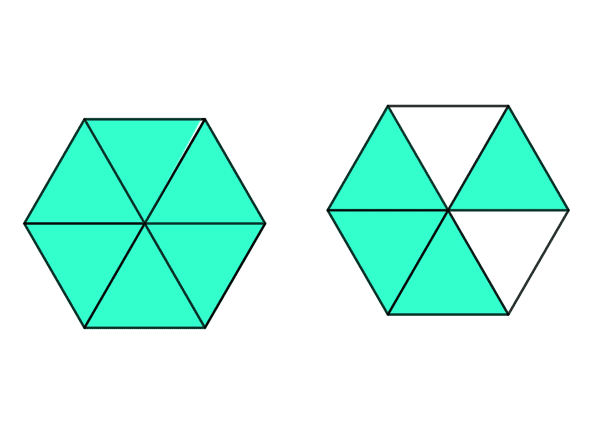•  Question 2: 1 pts The fraction that represents the part of the object that has been shaded is $\dfrac{6}{16}$.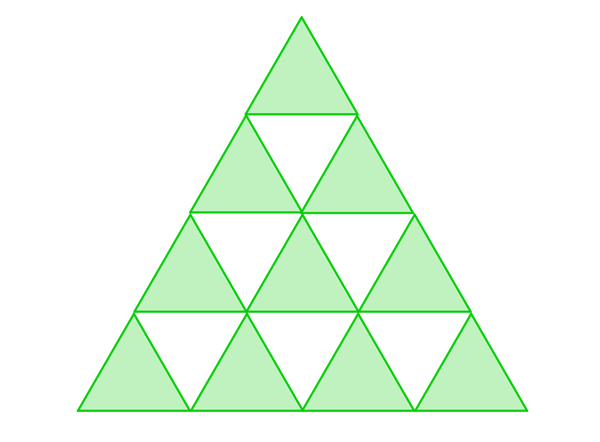•  Question 3: 2 pts Find the mixed number that corresponds to the shaded region.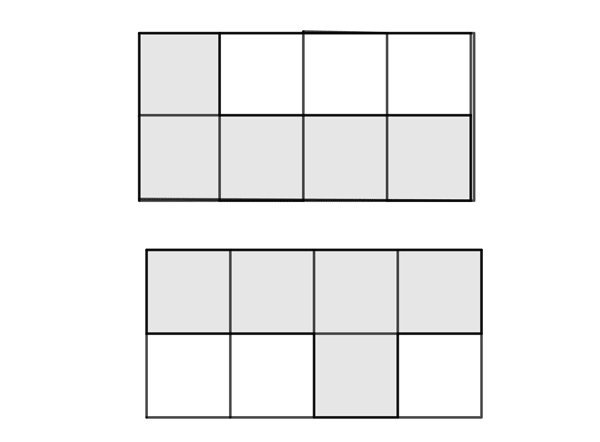$1\frac{1}{2}$ $\frac{9}{6}$ $1\frac{1}{6}$ $1\frac{1}{4}$
•  Question 4: 2 pts Find the improper fraction that corresponds to the shaded region.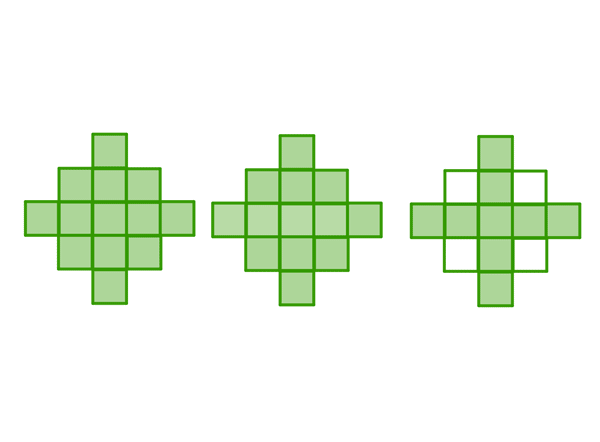$\frac{34}{13}$ $\frac{35}{13}$ $\frac{30}{13}$ $\frac{13}{30}$
•  Question 5: 2 pts Find the mixed number and the improper fraction that corresponds to the picture: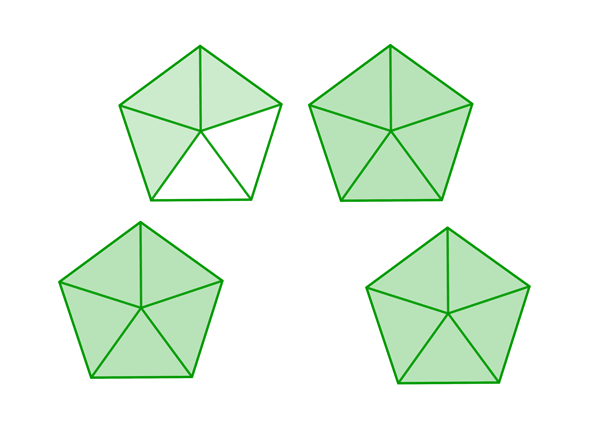•  Question 6: 2 pts Find the mixed number and the improper fraction that corresponds to the picture.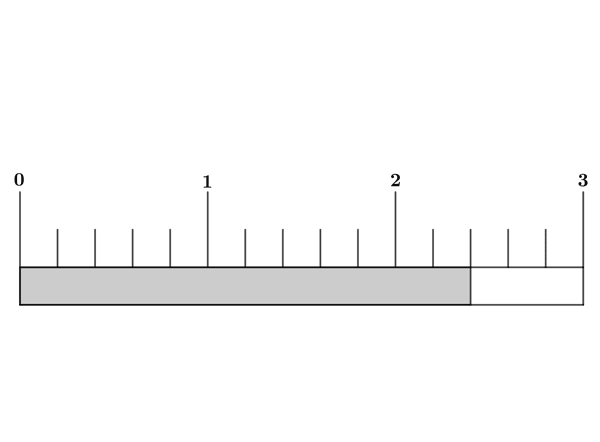•  Question 7: 3 pts Find the mixed number that corresponds to the shaded region.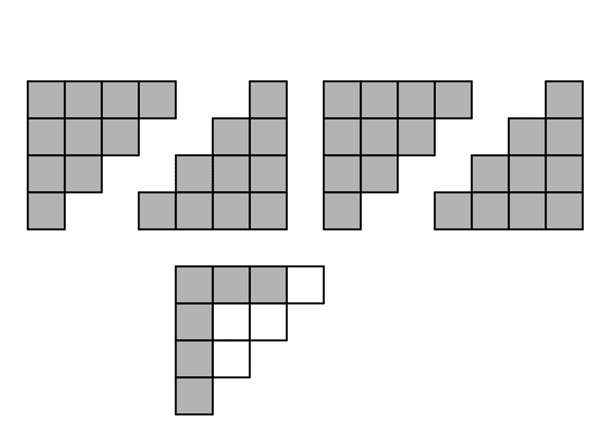$\frac{23}{5}$ $\frac{5}{23}$ $4\frac{3}{5}$ $4\frac{5}{3}$
•  Question 8: 3 pts Find the mixed number that corresponds to the shaded region.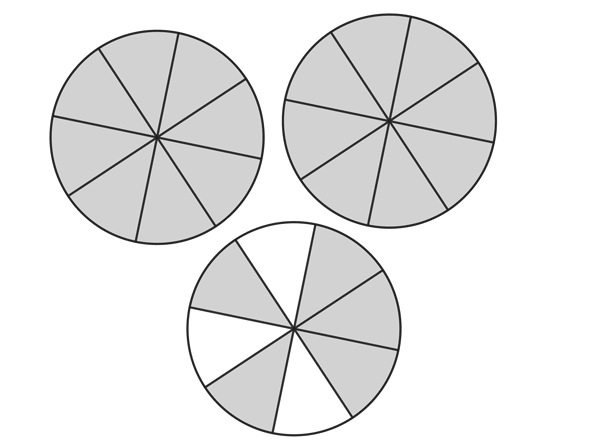$2\frac{8}{5}$ $2\frac{5}{8}$ $1\frac{3}{5}$ $4\frac{5}{8}$
•  Question 9: 3 pts The mixed number shown on the picture is $$2\frac{1}{4}.$$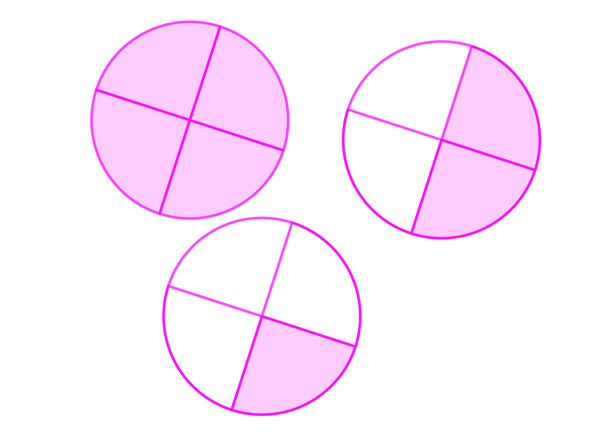•  Question 10: 3 pts Find the improper fraction that corresponds to the shaded region.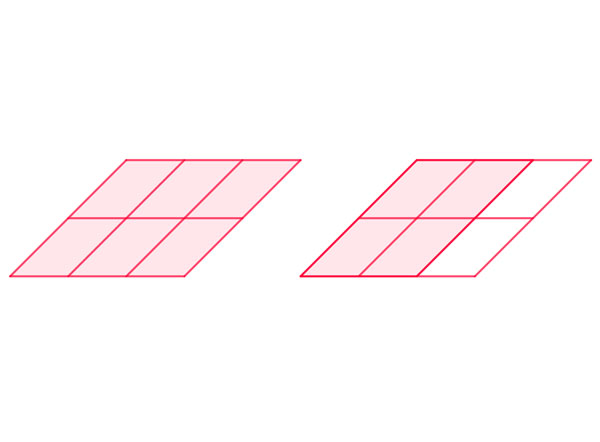$1\frac{4}{6}$ $\frac{5}{3}$ $1\frac{6}{4}$ $\frac{3}{5}$
•  Question 11: 3 pts Find the fraction that represents the part of the object that has been shaded.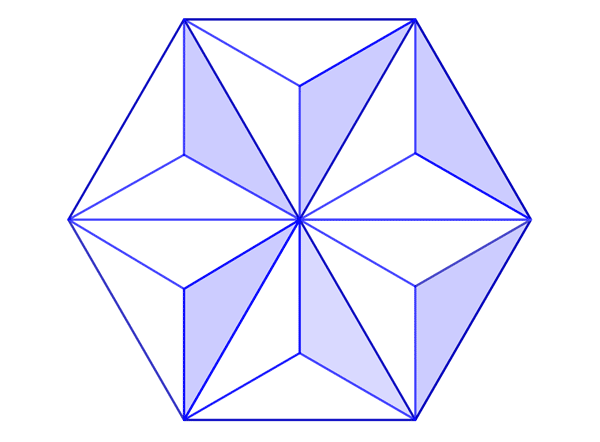$\dfrac{12}{18}$ $\dfrac{18}{12}$ $\dfrac{6}{18}$ $\dfrac{18}{6}$